# NCERT Solutions for Class 9 Maths Chapter 8 Linear Equations in Two Variables Ex 8.3

NCERT Solutions for Class 9 Maths Chapter 8 Linear Equations in Two Variables Ex 8.3 are part of NCERT Solutions for Class 9 Maths. Here we have given NCERT Solutions for Class 9 Maths Chapter 8 Linear Equations in Two Variables Ex 8.3.

 Board CBSE Textbook NCERT Class Class 9 Subject Maths Chapter Chapter 8 Chapter Name Linear Equations in Two Variables Exercise Ex 8.3 Number of Questions Solved 8 Category NCERT Solutions

## NCERT Solutions for Class 9 Maths Chapter 8 Linear Equations in Two Variables Ex 8.3

Question 1.
Draw the graph of each of the following linear equations in two variables
(i) x + y = 4
(ii) x – y = 2
(iii) y = 3x
(iv) 3 = 2x + y
Solution:
(i) x + y = 4
To draw the graph, we need atleast two solutions of the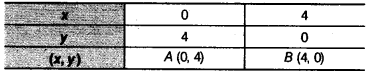So, draw the graph by plotting the two points from table and then joining by a line.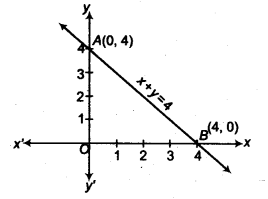(ii) x – y = 2
To draw the graph, we need atleast two solutions of the equation.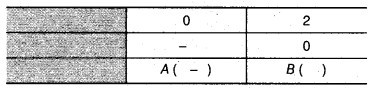So, draw the graph by plotting the two points from table and then joining by a line.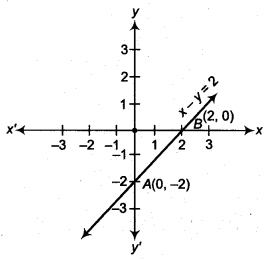(iii) y = 3x
To draw the graph, we need atleast two solutions of the equation.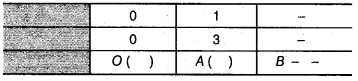So, draw the graph by plotting the two points from table and then by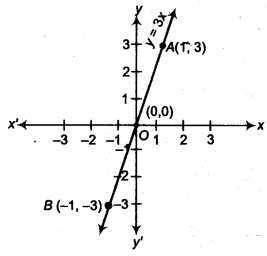(iv) 3 = 2x + y
To draw the graph, we need atleast two solutions of the equation.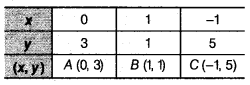So, draw the graph by plotting the points from the table and the by joining the same by a line.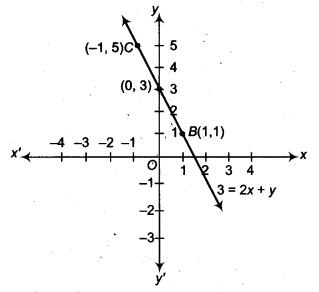Question 2.
Give the equations of two lines passing through (2,14). How many more such lines are there, and why?
Solution:
Here, (2,14) is a solution of a linear equation. One example of such a linear equation is y = 7x, others are x + y- 16.
There are infinitely many lines because there are infinitely many linear equations which are satisfied by the. coordinates of the point (2,14).

Question 3.
If the point (3,4) lies on the graph of the equation 3y – ax – 7, find the value of a.
Solution:
If the point (3, 4) lies on the graph, then it will satisfies the equations.
Hence, 3 (4) – a (3) – 7 = 0
⇒ 12 – 3a – 7 = 0
⇒ 5 – 3a = 0
⇒ 3a = 5
⇒ a = $$\frac { 5 }{ 3 }$$

Question 4.
The taxi fare in a city is as follows :
For the first kilometre, the fare is ₹ 8 and for the subsequent distance it is ₹5 per km. Taking the distance covered as x km and the total fare as ₹y, write a linear equation for this information and draw its graph.
Solution:
Distance covered = x km = 1+ (x – 1) km
Fare for first kilometre = ₹ 8
Fare for next (x-1 ) km = (x – 1) x 5 = 5(x-1)
According to question, total fare = y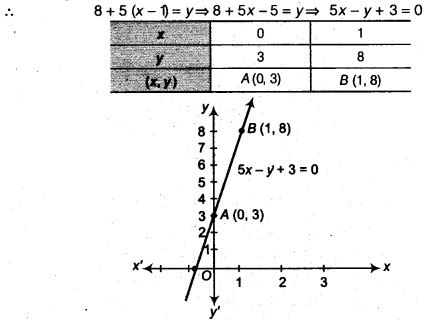Now, plot the points A (0,3) and B (1, 8) on a graph paper and joining them, to form q line AB.

Question 5.
From the choices given below, choose the equation whose graphs are given in Fig. (a) and Fig. (b).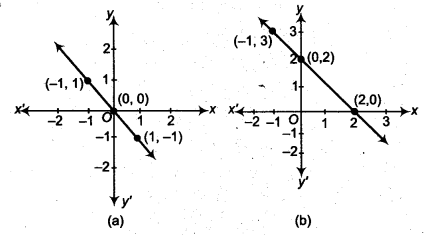For Fig. (a)
(i) y = x
(ii) x + y = 0
(iii) y = 2x
(iv) 2 + 3y = 7x

For Fig. (b)
(i) y = x + 2
(ii) y = x – 2
(iii) y = – x + 2
(iv) x + 2y = 6
Solution:
In Fig. (a), we observe that the points (-1,1) and (1,-1) passes through the equation x + y = 0
∵ At (-1,1) x + y = -1 + 1 = 0
and at (1, -1) x + y = 1 -1 = 0
In Fig. (b), we observe that the points (-1, 3), (0, 2) and (2, 0) passes through the equation x + y = 2.
∵ At (-1,3), x + y = -1 + (3) = + 2
At ( 0 , 2 ) , x +y = 0+2 = 2
and at ( 2, 0 ) x+ y = 2 + 0= 2

Question 6.
If the work done by a body on application of a constant force is directly proportional to the distance travelled by the body, express this in the form of an equation in two variables and draw the graph of the same by taking the constant force as 5 units. Also, read from the graph the work done when the distance travelled by the body is.
(i) 2 units
(ii) 0 unit.
Solution:
Given: that, work done by a body on application of a constant force is directly proportional to the distance travelled by the body.
i.e., (W) work done ∝ distance (s)
⇒ W = F. s
(Where, F = arbitrary constant which take the value 5 units)
∴ W = 5s …(i)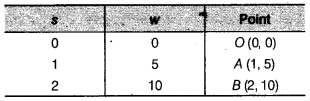Now, plot the points O (0,0), A (1,5) and B (2,10) on graph paper and joining them to get a line AB.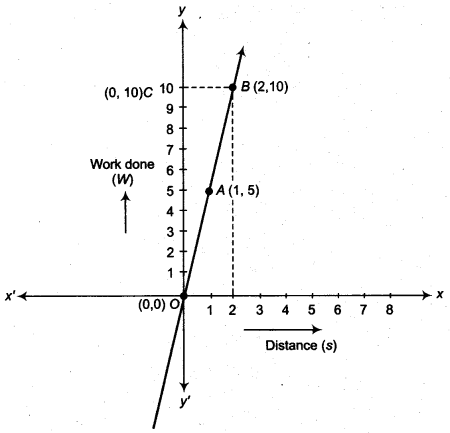(i) From point B (2,10), draw a line parallel to OY to intersect the x-axis at (2,0) and draw a line parallel to x-axis intersect at C (0, 10).
∴ Work done when the distance travelled by the body is 2 units = 10units.
(ii) Clearly y = 0 when x = 0 so the work done when the distance travelled by the body is 0 unit.

Question 7.
Yamini and Fatima, two students of class IX of a school, together contributed ₹100 towards the Prime Minister’s Relief Fund to help the earthquake victims. Write a linear equation which satisfies this data. (You may take their contributions as ₹ x and ₹ y.) Draw the graph of the same.
Solution:
Let the contributions of Yamini and Fatima together towards the Prime Minister’s Relief Fund to help the earthquake victims are ₹x and ₹ y, respectively.
Then, by given condition,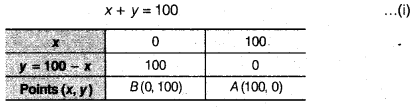Here, we plot the points B (0,100) and A (100, 0) on graph paper and join all these points to form a line AB.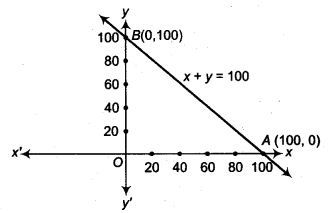Question 8.
In countries like USA and Canada, temperature is measured in Fahrenheit, whereas in countries like India, it is measured in Celsius. Here is a linear equation that converts Fahrenheit to Celsius.
F = ($$\frac { 9 }{ 5 }$$ ) c + 32
(i) Draw the graph of the linear equation above using Celsius for x-axis and Fahrenheit for y-axis.
(ii) If the temperature is 30 °C, what is the temperature in Fahrenheit?
(iii) If the temperature is 95°F, what is the temperature in Celsius?
(iv) If the temperature is 0°C, what is the temperature in Fahrenheit and if the temperature is 0°F, what is the temperature in Celsius?
(v) Is there a temperature which is numerically the same in both Fahrenheit and Celsius? If yes, find it.
Solution:
(i) Given, linear equation in Fahrenheit and Celsius is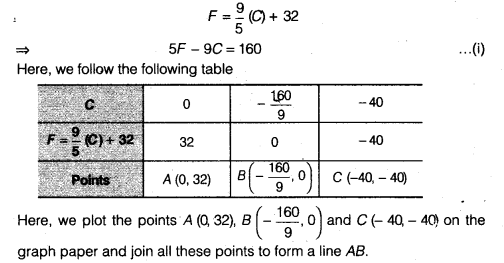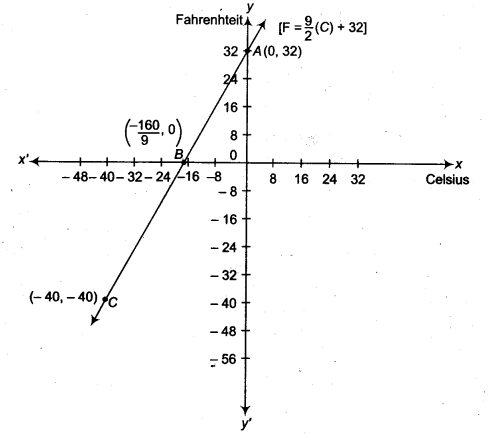(ii) If temperature is 30°C i.e., C = 30°C
Then, from Eq. (i), we get
F = $$\frac { 9 }{ 5 }$$ x 30 + 32 = 9 x 6 + 32 = 54 + 32 = 86
∴ Temperature in Fahrenheit = 86°F

(iii) If temperature is 95°F i.e., F= 95°F
Then, from Eq. (i),we get
5 x 95 – 9C = 160 ⇒ 9C= 475 – 160= 315 ⇒ C= 35°
∴ Temperature in Celsius = 35°C

(iv) If the temperature is 0°C i.e., C= 0
Then, from Eq. (i), we get
F = $$\frac { 9 }{ 5 }$$ x 0 + 32 = -32
∴ Temperature in Fahrenheit = 32°F
If the temperature is 0°F i.e., F = 0
Then, from Eq. (i), we get
5 x 0 – 9C = 160
⇒ C = $$\frac { -160 }{ 9 }$$ = – 17.8° C (Approx.)
∴ Temperature in Celsius = -17.8° C

(v) Yes, if we take both temperature are equal i.e., C = F.
Now, from Eq. (i), we get
F = $$\frac { 9 }{ 5 }$$ F + 32
⇒ 5F = 9F + 160
⇒ -4F = 160
⇒ F = -40°
∴ F = C = – 40°
We hope the NCERT Solutions for Class 9 Maths Chapter 8 Linear Equations in Two Variables Ex 8.3 help you. If you have any query regarding NCERT Solutions for Class 9 Maths Chapter 8 Linear Equations in Two Variables Ex 8.3, drop a comment below and we will get back to you at the earliest.